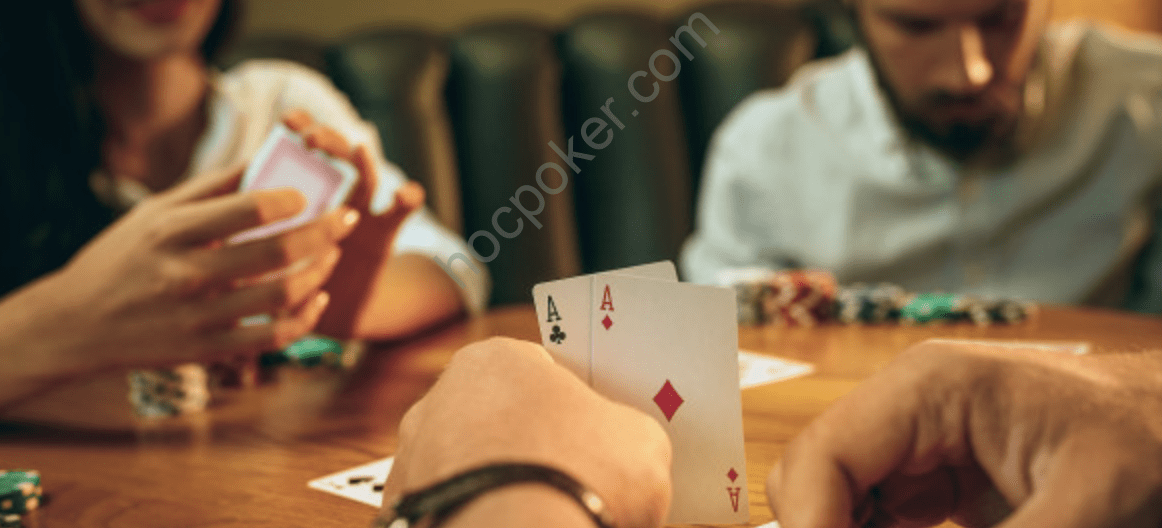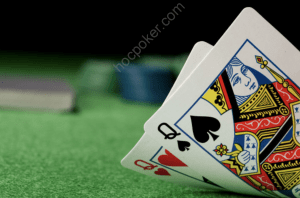# Poker MathExpected Value (EV)

In poker, expected value (EV) is a frequently used term. It means how much you are expected to win in a certain situation on average. An EV calculation is simply an actual math calculation to figure out more exactly what someone’s \$EV (their real money expectation) is in a pot. EV calculations are good practice, and are well worth carry‐ ing out to increase your learning. However, they are important for a better reason – they are a mathematical way of writing out what is really happening in every hand of poker, whether it’s thought about in these terms or not.

Basic probability

Let’s start with some basic math – for example the odds of hitting a flush draw. There are 52 cards in a deck, five of which are visible af‐ ter the flop – the three on the board and the two in your hand. There are either two flush cards in your hand and two on the board, or three on the board and one on your hand. That means there are nine

flush cards left in the deck out of 47 unseen cards. The odds of one coming on the turn is simply 9/47. The odds of two flush cards com‐ ing consecutively is 9/47 * 8/46. The odds of one flush card coming and one non flush card is 9/47 * 38/46. The odds of no flush card coming twice is 38/47 * 37/46.

We can’t do such multiplications in real time so a good way to calcu‐ late the odds if we are considering a “two street scenario” is to esti‐ mate based on a few facts. With six outs and two cards to come (e.g. a two overcard draw) the odds of it hitting are slightly worse than 3‐ 1, i.e. it will happen 1/4 times. The odds of a nine‐outer coming (e.g. a flush draw) is a little better than 2‐1 or 1/3 times. And the odds of a 12‐outer hitting (e.g. a flush draw and overcard) is almost even money. These numbers can be used as a point of reference, so if the number of outs is somewhere near these numbers, the probability can be estimated by raising or lowering one of these memorized ex‐ amples as needed.

When counting outs, runner‐runner flush draws and straight draws are also worth considering. Say the board is 5♥‐6♥‐9♦ and I have A♥-2♣, the odds that the flush comes are 10/47 * 9/46 = 0.042, which adds about 4% to the chances of winning (which is roughly equiva‐ lent to an additional out). As will be seen later on, simply picking up the flush draw on the turn (regardless of whether it hits on the river) can be a very powerful weapon, allowing us to bluff and be aggres‐ sive. Similarly with a straight, if the board is 3‐4‐10 and I have K‐Qo, I need a jack to come and then either a 9 or an ace so that is 4/47 * 8/46 * 2 = 0.03 or about 3%.Previous post MultitablingNext post Hand Combinations